The VARMAX Procedure

OUTEST= Data Set

The OUTEST= data set contains estimation results of the fitted model produced by the VARMAX statement. The following output variables can be created:

• BY variables

• NAME, a character variable that contains the name of the endogenous (dependent) variables or the name of the parameters for the covariance of the matrix of the parameter estimates if you specify the OUTCOV option

• TYPE, a character variable that contains the value EST for parameter estimates, the value STD for standard error of parameter estimates, and the value COV for the covariance of the matrix of the parameter estimates if you specify the OUTCOV option

• CONST, a numeric variable that contains the estimates of constant parameters and their standard errors

• SEASON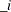, a numeric variable that contains the estimates of seasonal dummy parameters and their standard errors, where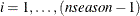, andis based on the NSEASON= option

• LTREND, a numeric variable that contains the estimates of linear trend parameters and their standard errors

• QTREND, a numeric variable that contains the estimates of quadratic trend parameters and their standard errors

• XL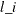, numeric variables that contain the estimates of exogenous parameters and their standard errors, where l is the lag lth coefficient matrix and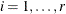, where r is the number of exogenous variables

• AR, numeric variables that contain the estimates of autoregressive parameters and their standard errors, where l is the lag lth coefficient matrix and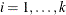, where k is the number of endogenous variables

• MA, numeric variables that contain the estimates of moving-average parameters and their standard errors, where l is the lag lth coefficient matrix and, where k is the number of endogenous variables

• COV, numeric variables that contain the estimates of the covariance of innovations parameters when the maximum likelihood method is applied, where• DCCAB, a numeric variable that contains the estimates ofor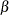in the correlation equation for DCC representation and their standard errors

• CCC, numeric variables that contain the estimates of the conditional constant correlation parameters for CCC representation, where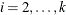• DCCS, numeric variables that contain the estimates of the unconditional correlation parameters for DCC representation, where• GCHC, numeric variables that contain the estimates of the constant parameters of the covariance matrix and their standard errors, wherefor BEKK representation, k is the number of endogenous variables, and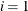for CCC and DCC representations

• ACH, numeric variables that contain the estimates of the ARCH parameters of the covariance matrix and their standard errors, where l is the lag lth coefficient matrix andfor BEKK, CCC, and DCC representations, where k is the number of endogenous variables

• EACH, numeric variables that contain the estimates of the exponential ARCH parameters of the covariance matrix and their standard errors, where l is the lag lth coefficient matrix andfor CCC and DCC representations, where k is the number of endogenous variables

• PACH, numeric variables that contain the estimates of the power ARCH parameters of the covariance matrix and their standard errors, where l is the lag lth coefficient matrix andfor CCC and DCC representations, where k is the number of endogenous variables

• QACH, numeric variables that contain the estimates of the quadratic ARCH parameters of the covariance matrix and their standard errors, where l is the lag lth coefficient matrix andfor CCC and DCC representations, where k is the number of endogenous variables

• TACH, numeric variables that contain the estimates of the threshold ARCH parameters of the covariance matrix and their standard errors, where l is the lag lth coefficient matrix andfor CCC and DCC representations, where k is the number of endogenous variables

• GCH, numeric variables that contain the estimates of the GARCH parameters of the covariance matrix and their standard errors, where l is the lag lth coefficient matrix andfor BEKK, CCC, and DCC representations, where k is the number of endogenous variables

• LAMBDA, a numeric variable that contains the estimates of power parameters in the PGARCH model for CCC and DCC representations and their standard errors

The OUTEST= data set contains the values shown in Table 35.9 for a bivariate case.

Table 35.9: OUTEST= Data Set

 Obs NAME TYPE CONST AR1_1 AR1_2 AR2_1 AR2_2 1 y1 EST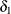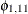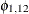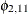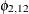2 STD se() se() se() se() se() 3 y2 EST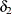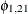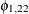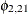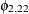4 STD se() se() se() se() se()

Consider the following example:

proc varmax data=simul2 outest=est;
model y1 y2 / p=2 noint
ecm=(rank=1 normalize=y1)
noprint;
run;

proc print data=est;
run;


The output in Figure 35.67 shows the results of the OUTEST= data set.

Figure 35.67: OUTEST= Data Set

Obs NAME TYPE AR1_1 AR1_2 AR2_1 AR2_2
1 y1 EST -0.46680 0.91295 -0.74332 -0.74621
2   STD 0.04786 0.09359 0.04526 0.04769
3 y2 EST 0.10667 -0.20862 0.40493 -0.57157
4   STD 0.05146 0.10064 0.04867 0.05128